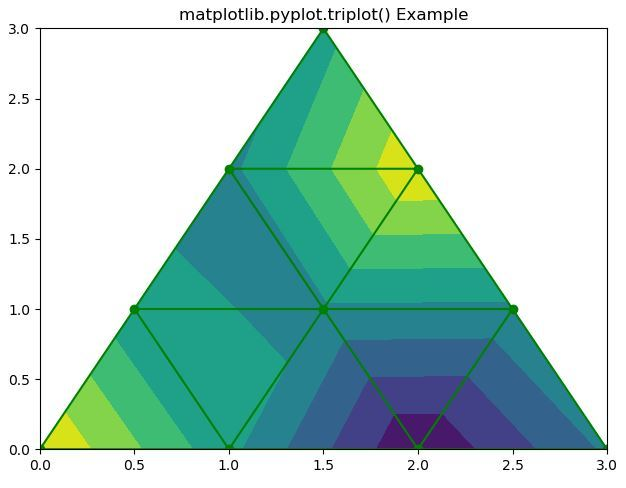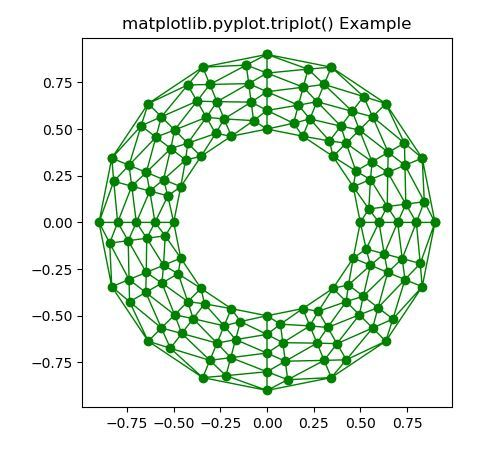Skip to content
Related Articles
Matplotlib.pyplot.triplot() in python
• Last Updated : 19 Apr, 2020

Matplotlib is a library in Python and it is numerical – mathematical extension for NumPy library. Pyplot is a state-based interface to a Matplotlib module which provides a MATLAB-like interface. There are various plots which can be used in Pyplot are Line Plot, Contour, Histogram, Scatter, 3D Plot, etc.

matplotlib.pyplot.triplot() Function:

The triplot() function in pyplot module of matplotlib library is used to draw a unstructured triangular grid as lines and/or markers.

Syntax: matplotlib.pyplot.triplot(*args, **kwargs)

Parameters: This method accept the following parameters that are described below:

• x, y: These parameter are the x and y coordinates of the data which is to be plot.
• triangulation: This parameter is a matplotlib.tri.Triangulation object.
• **kwargs: This parameter is Text properties that is used to control the appearance of the labels.

All remaining args and kwargs are the same as for matplotlib.pyplot.plot().

Returns: This returns the list of 2 Line2D containing following:

• The lines plotted for triangles edges.
• The markers plotted for triangles nodes

Below examples illustrate the matplotlib.pyplot.triplot() function in matplotlib.pyplot:

Example 1:

 `# Implementation of matplotlib function``import` `matplotlib.pyplot as plt``import` `matplotlib.tri as mtri``import` `numpy as np``  ` `# Create triangulation.``x ``=` `np.asarray([``0``, ``1``, ``2``, ``3``, ``0.5``, ``1.5``,``                ``2.5``, ``1``, ``2``, ``1.5``])`` ` `y ``=` `np.asarray([``0``, ``0``, ``0``, ``0``, ``1.0``,``                ``1.0``, ``1.0``, ``2``, ``2``, ``3.0``])`` ` `triangles ``=` `[[``0``, ``1``, ``4``], [``1``, ``2``, ``5``], ``             ``[``2``, ``3``, ``6``], [``1``, ``5``, ``4``], ``             ``[``2``, ``6``, ``5``], [``4``, ``5``, ``7``],``             ``[``5``, ``6``, ``8``], [``5``, ``8``, ``7``],``             ``[``7``, ``8``, ``9``]]`` ` `triang ``=` `mtri.Triangulation(x, y, triangles)``z ``=` `np.cos(``1.5` `*` `x) ``*` `np.cos(``1.5` `*` `y)``  ` `plt.tricontourf(triang, z)``plt.triplot(triang, ``'go-'``)``  ` `plt.title(``'matplotlib.pyplot.triplot() Example'``)``plt.show()`

Output:Example 2:

 `# Implementation of matplotlib function``import` `matplotlib.pyplot as plt``import` `matplotlib.tri as tri``import` `numpy as np``  ` `n_angles ``=` `24``n_radii ``=` `9``min_radius ``=` `0.5``radii ``=` `np.linspace(min_radius, ``0.9``,``                    ``n_radii)``  ` `angles ``=` `np.linspace(``0``, ``3` `*` `np.pi, n_angles, ``                     ``endpoint ``=` `False``)`` ` `angles ``=` `np.repeat(angles[..., np.newaxis], ``                   ``n_radii, axis ``=` `1``)`` ` `angles[:, ``1``::``2``] ``+``=` `np.pi ``/` `n_angles``  ` `x ``=` `(radii ``*` `np.cos(angles)).flatten()``y ``=` `(radii ``*` `np.sin(angles)).flatten()``triang ``=` `tri.Triangulation(x, y)``  ` `triang.set_mask(np.hypot(x[triang.triangles].mean(axis ``=` `1``),``                         ``y[triang.triangles].mean(axis ``=` `1``))``                ``< min_radius)`` ` `plt.triplot(triang, ``'go-'``, lw ``=` `1``)``plt.title(``'matplotlib.pyplot.triplot() Example'``)``plt.show()`

Output:Attention geek! Strengthen your foundations with the Python Programming Foundation Course and learn the basics.

To begin with, your interview preparations Enhance your Data Structures concepts with the Python DS Course. And to begin with your Machine Learning Journey, join the Machine Learning – Basic Level Course

My Personal Notes arrow_drop_up